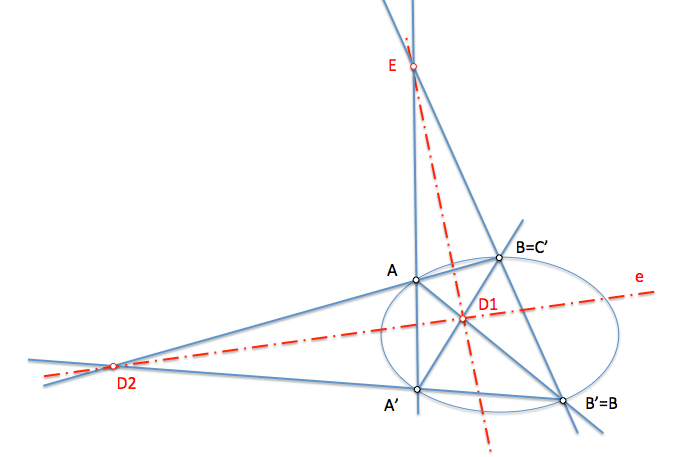Geometría proyectiva: Conjugate polar diametersWe have seen the definition of polar conjugate diameters, given to analyze the concept of Conjugate directions:

Conjugate polar diameters: They are polar two conjugated improper point.

Let's see how we can relate this concept with the of autopolar triangle seen in Involutions in second-order series.

To establish an involution in a conical between two pairs of points, We got a Center of involution (And) and axis of involution (and) He related them. Each pair of homologous points, A-A’, they were aligned with the Center and involution and projecting from them any pair of homologous elements, these rays, perspective, they were cut in the axis of involution.

In this transformation the two pairs of homologous points determining a full cuadrivertice, being involution Center and one of its diagonal points (D3), While them other two (D1 and D2) about shaft of involution.

Three diagonal points determining a autopolar triangle, as the polar of each one of them was determined by the opposite side containing the other two.If the diagonal D2 point at infinity, the polar line of this point (straight E-D1) It passes through the midpoints of strings containing D2, ropes parallel to a-b, TO ’-B’ etc., Since the harmonic separation forces to this polar to determine shortlists with value -1 as we have seen when studying the Conjugate directions. Polar D2 will therefore contain the center of the Conic.If we move in Center of involution and the infinite, third point diagonal of triangle autopolar, the diagonal D1 point happens to coincide with the center of the Conic, Since is the pole of the improper line D2-D3 or D2-E .

D1-D2 and D1-D3 will be a pair of conjugate diameters, being the third side of the triangle autopolar the infinite line.